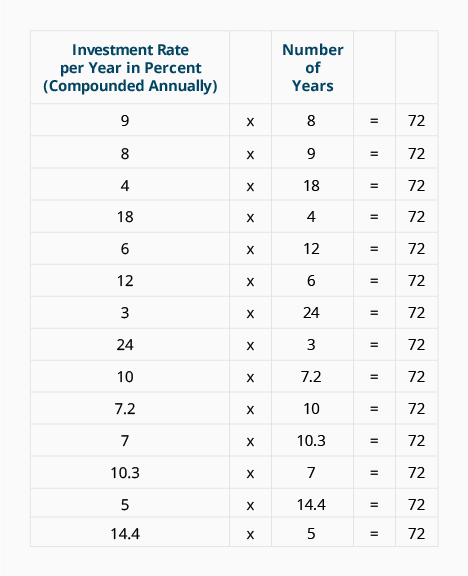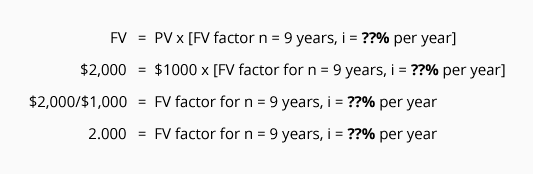# Double Your Money: The Rule of 72

The Rule of 72 is a quick and simple technique for estimating one of two things:

• The time it takes for a single amount of money to double with a known interest rate.
• The rate of interest you need to earn for an amount to double within a known time period.

The rule states that an investment or a cost will double when:

[Investment Rate per year as a percent] x [Number of Years] = 72.

When interest is compounded annually, a single amount will double in each of the following situations:The Rule of 72 indicates than an investment earning 9% per year compounded annually will double in 8 years. The rule also means if you want your money to double in 4 years, you need to find an investment that earns 18% per year compounded annually.

You can confirm the rationality of the Rule of 72 as follows: Find factors on the FV of 1 Table that are close to 2.000. (The factor of 2.000 tells you that the present value of 1.000 had doubled to the future value of 2.000.) When you find a factor close to 2.000, look at the interest rate at the top of the column and look at the number of periods (n) in the far left column of the row containing the factor. Multiply that interest rate times the number of periods and you will get the product 72.

To use the Rule of 72 in order to determine the approximate length of time it will take for your money to double, simply divide 72 by the annual interest rate. For example, if the interest rate earned is 6%, it will take 12 years (72 divided by 6) for your money to double. If you want your money to double every 8 years, you will need to earn an interest rate of 9% (72 divided by 8).

Here's another way to demonstrate that the Rule of 72 works. Assume you make a single deposit of \$1,000 to an account and wish for it to grow to a future value of \$2,000 in nine years. What annual interest rate compounded annually will the account have to pay? The Rule of 72 indicates that the rate must be 8% (72 divided by 9 years). Let's verify the rate with the format we used with the FV Table:To finish solving the equation, we search only the "n = 9" row of the FV of 1 Table for the FV factor that is closest to 2.000. The factor closest to 2.000 in the row where n = 9 is 1.999 and it is in the column where i = 8%. An investment at 8% per year compounded annually for 9 years will cause the investment to double (8 x 9 = 72).